Proving Statements true or false

Mathematical Reasoning
Serial order wise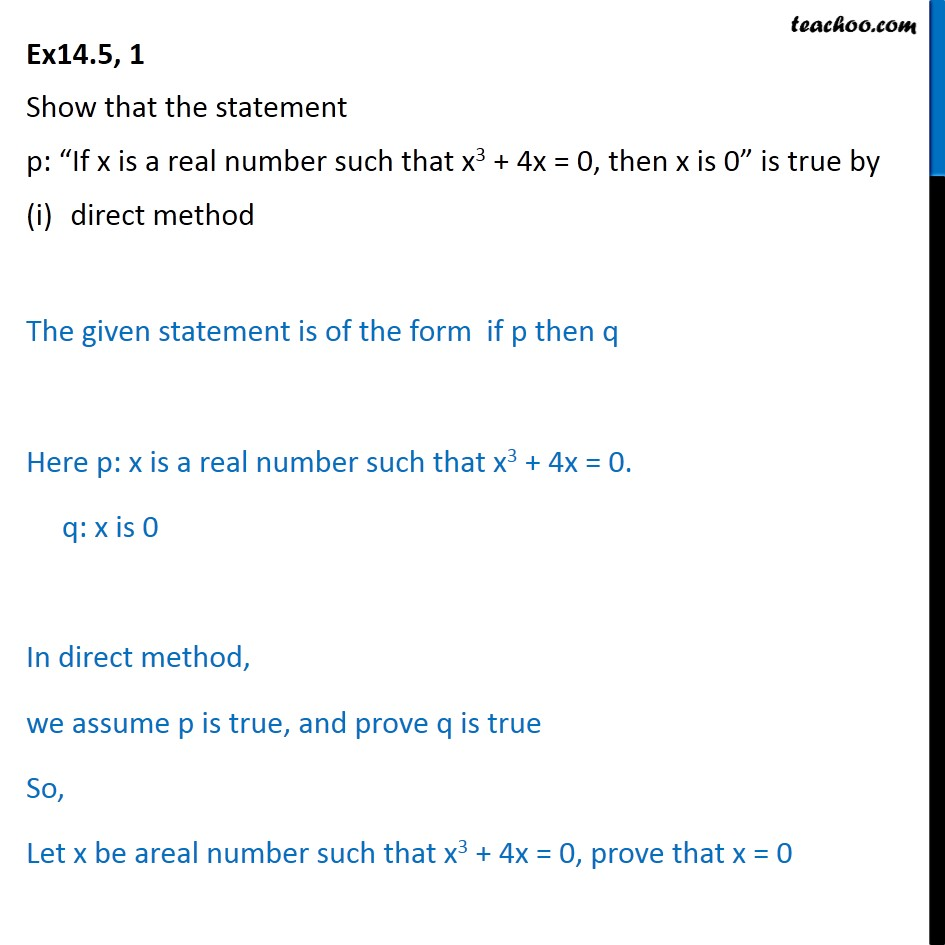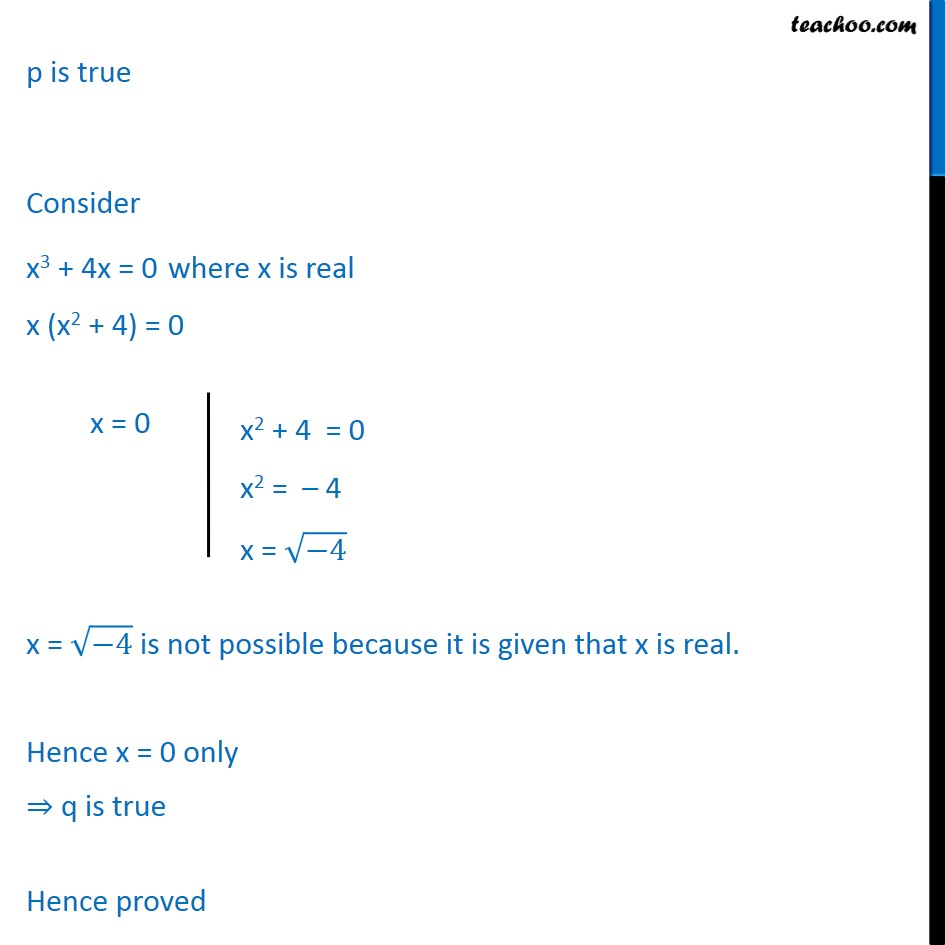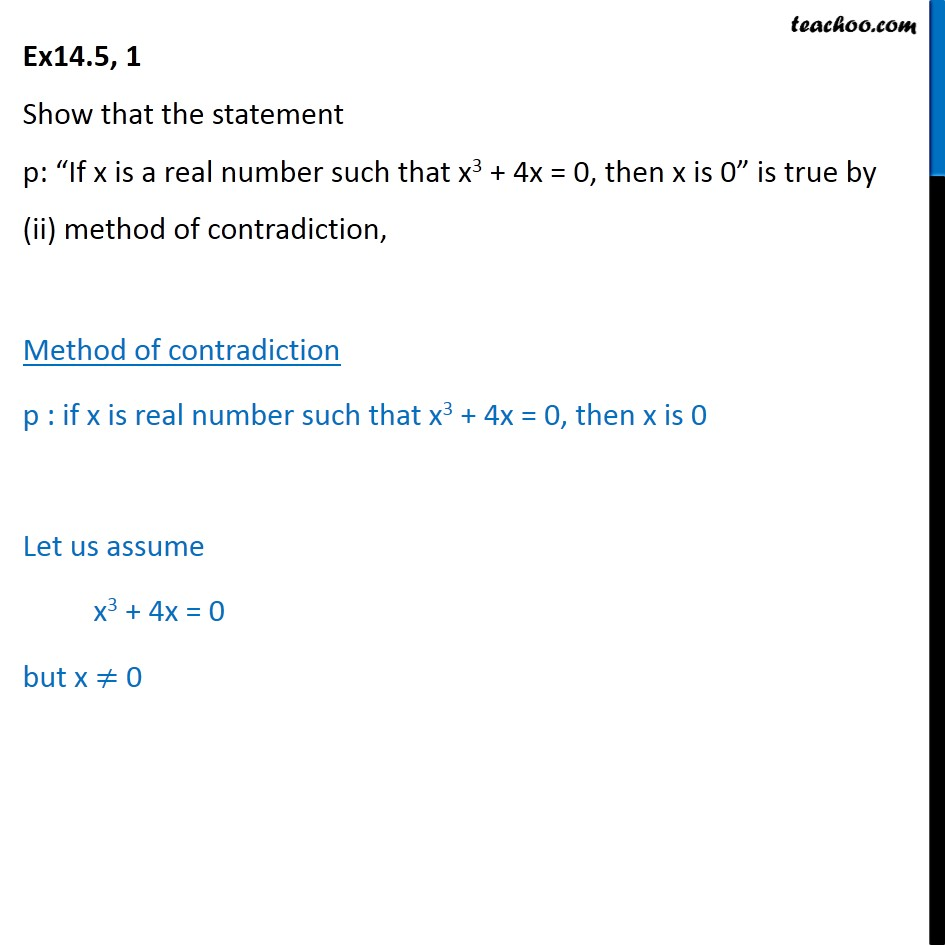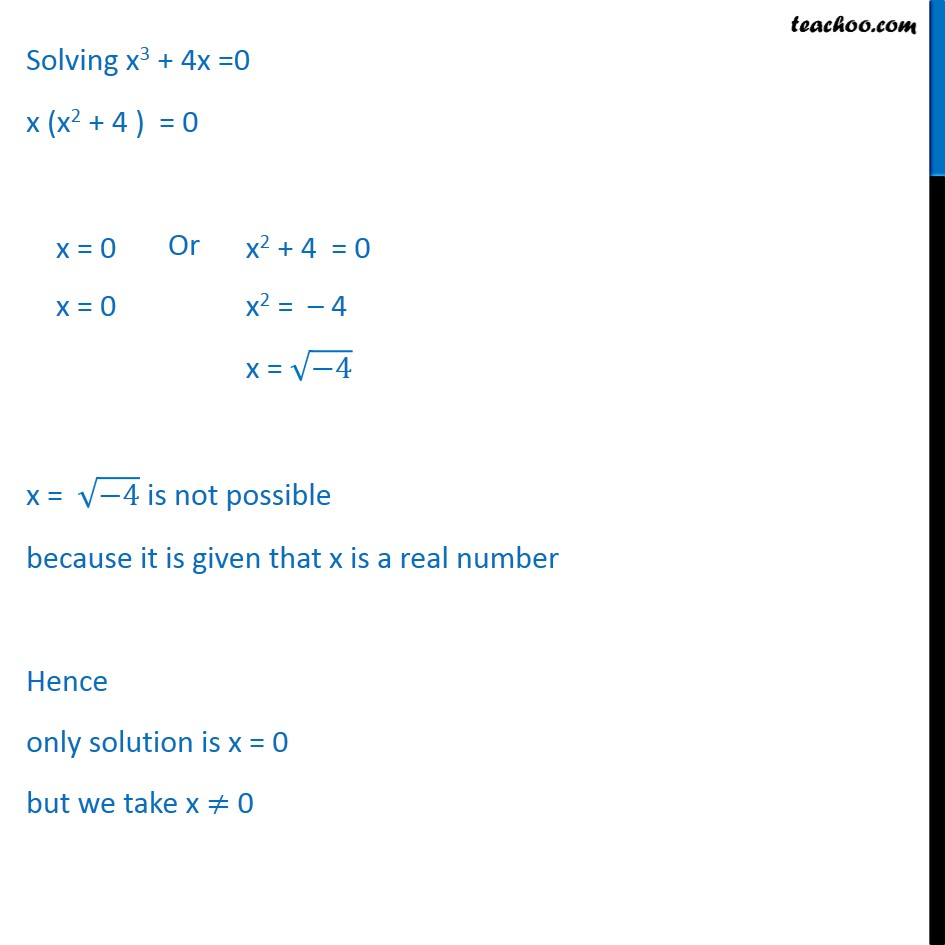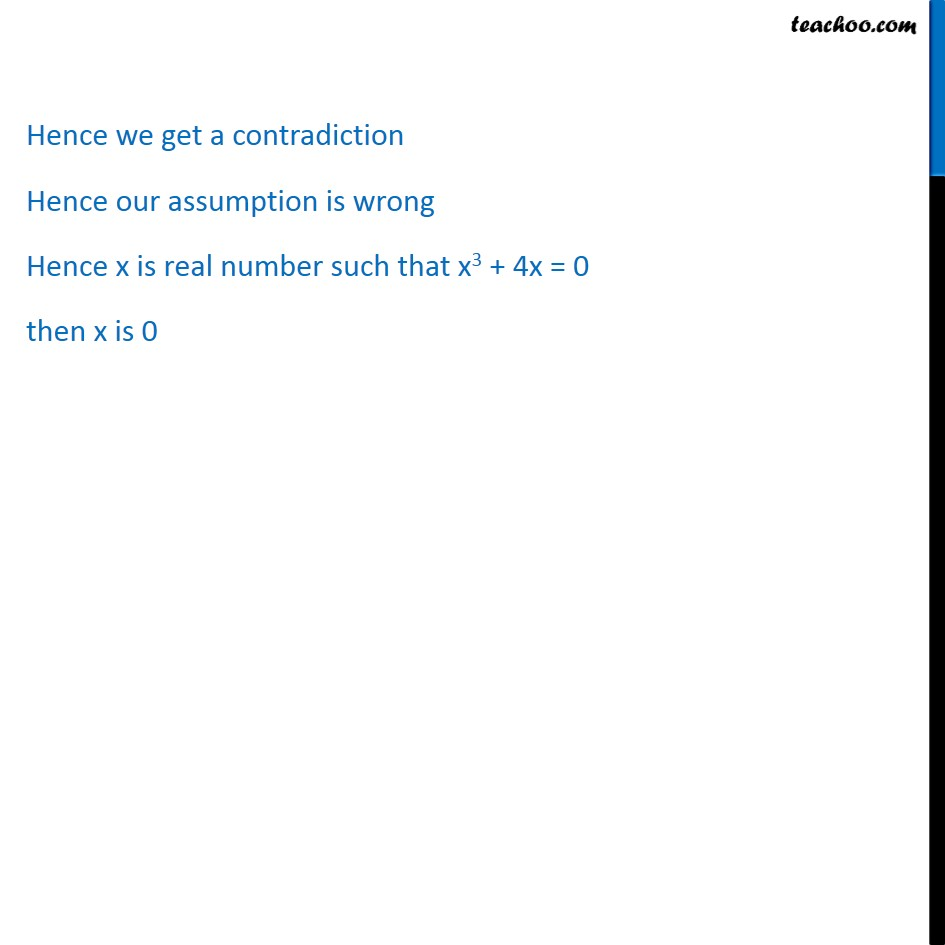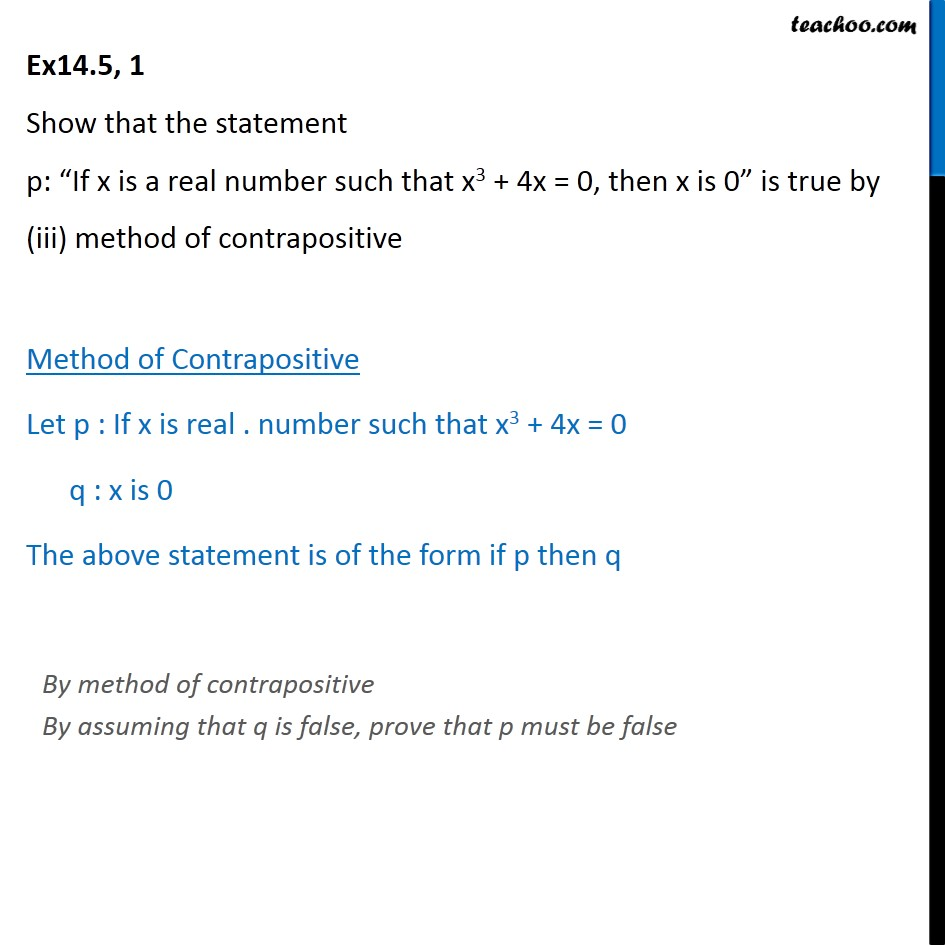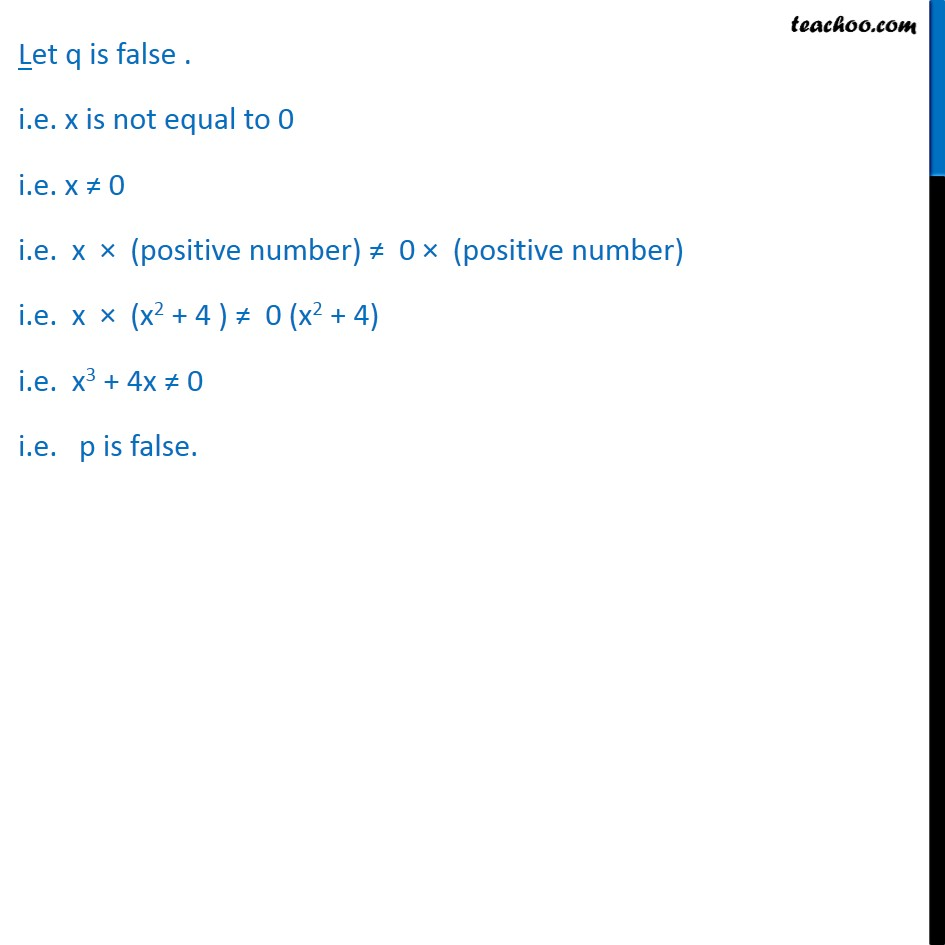Learn in your speed, with individual attention - Teachoo Maths 1-on-1 Class

### Transcript

Question 1 Show that the statement p: If x is a real number such that x3 + 4x = 0, then x is 0 is true by direct method The given statement is of the form if p then q Here p: x is a real number such that x3 + 4x = 0. q: x is 0 In direct method, we assume p is true, and prove q is true So, Let x be areal number such that x3 + 4x = 0, prove that x = 0 p is true Consider x3 + 4x = 0 where x is real x (x2 + 4) = 0 x = 4 is not possible because it is given that x is real. Hence x = 0 only q is true Hence proved Ex14.5, 1 Show that the statement p: If x is a real number such that x3 + 4x = 0, then x is 0 is true by (ii) method of contradiction, Method of contradiction p : if x is real number such that x3 + 4x = 0, then x is 0 Let us assume x3 + 4x = 0 but x 0 Solving x3 + 4x =0 x (x2 + 4 ) = 0 Or x = 4 is not possible because it is given that x is a real number Hence only solution is x = 0 but we take x 0 Hence we get a contradiction Hence our assumption is wrong Hence x is real number such that x3 + 4x = 0 then x is 0 Ex14.5, 1 Show that the statement p: If x is a real number such that x3 + 4x = 0, then x is 0 is true by (iii) method of contrapositive Method of Contrapositive Let p : If x is real . number such that x3 + 4x = 0 q : x is 0 The above statement is of the form if p then q Let q is false . i.e. x is not equal to 0 i.e. x 0 i.e. x (positive number) 0 (positive number) i.e. x (x2 + 4 ) 0 (x2 + 4) i.e. x3 + 4x 0 i.e. p is false.